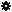﻿ Object Model: PolygonFace.NeighborEdges

PolygonFace.NeighborEdges

## PolygonFace.NeighborEdgesv3.0

### Description

Returns a collection of Edge neighbors within a given distance. If the distance is 1 all Edge objects comprising the current PolygonFace are returned in counter-clockwise order.

### C# Syntax

 ```EdgeCollection PolygonFace.NeighborEdges( Int32 in_lDistance ); ```

### Scripting Syntax

 ```oReturn = PolygonFace.NeighborEdges( [Distance] ); ```

### Parameters

Parameter Type Description
Distance Integer Distance is an integer value representing the degree of neighborhood (eg: degree=2 for a polygon means its adjacent edges plus the adjacent edges of the adjacent polygons)

Default Value: 1

### Examples

#### VBScript Example

 ```set oCube = ActiveSceneRoot.AddGeometry( "Cube", "MeshSurface" ) set oGeometry = oCube.ActivePrimitive.Geometry for each Polygon in oGeometry.Polygons set oNeighbors = Polygon.NeighborEdges( 1 ) str = "Edges neighbors of polygon( " & Polygon.index & ") are :" for each edge in oNeighbors str = str & " " & edge.index next logmessage str next ```